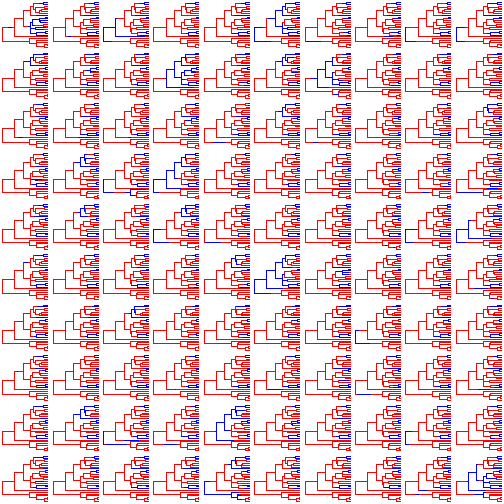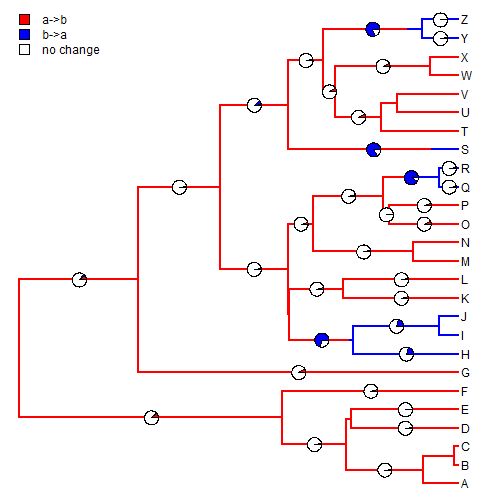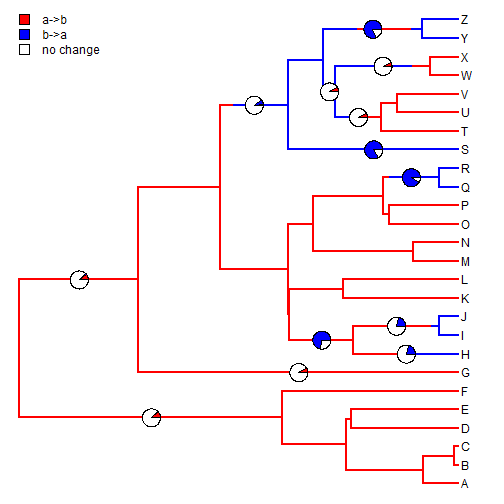## Wednesday, November 11, 2015

### Computing the relative frequencies of changes of different types along edges in a sample of stochastic map trees

I just added a function to phytools to compute the relative frequency of changes between states along edges in a sample of trees from stochastic mapping using `make.simmap`. This is similar to what I showed here, but with the added detail that the frequency of changes between all states are computed.

First, here is what the function looks like, as it is relatively simple:

``````edgeProbs <- function (trees)
{
if (!inherits(trees, "multiSimmap"))
stop("trees should be an object of class \"multiSimmap\".")
SS <- sapply(trees, getStates, "tips")
states <- sort(unique(as.vector(SS)))
m <- length(states)
TT <- sapply(states, function(x, y) sapply(y, paste, x, sep = "->"),
y = states)
nn <- c(TT[upper.tri(TT)], TT[lower.tri(TT)])
fn <- function(edge, trees, states) {
obj <- sapply(trees, function(x, e, s) if (names(x\$maps[[e]]) ==
s && names(x\$maps[[e]])[length(x\$maps[[e]])] ==
s)
TRUE
else FALSE, e = edge, s = states)
sum(obj)/length(obj)
}
edge.probs <- matrix(0, nrow(trees[]\$edge), m, dimnames = list(apply(trees[]\$edge,
1, paste, collapse = ","), nn))
k <- 1
for (i in 1:m) for (j in 1:m) {
if (i != j) {
edge.probs[, k] <- sapply(1:nrow(trees[]\$edge),
fn, trees = trees, states = states[c(i, j)])
k <- k + 1
}
}
edge.probs <- cbind(edge.probs, 1 - rowSums(edge.probs))
colnames(edge.probs)[ncol(edge.probs)] <- "no change"
edge.probs
}
``````

Now, let's see it in action. First, here are our trees:

``````trees
``````
``````## 100 phylogenetic trees with mapped discrete characters
``````

Let's plot them:

``````colors<-setNames(c("blue","red"),c("a","b"))
par(mfrow=c(10,10))
plot(trees,ftype="off",lwd=1,colors=colors)
``````Now let's use `edgeProbs` to compute the relative frequency of branches in which the mapped state changes from one value to another. Note that this only considers the states at flanking nodes, and does not count “multiple-hits” that occur along edges.

``````obj<-edgeProbs(trees)
obj
``````
``````##       a->b b->a no change
## 27,28 0.12 0.01      0.87
## 28,29 0.02 0.00      0.98
## 29,30 0.01 0.00      0.99
## 30,1  0.00 0.00      1.00
## 30,31 0.00 0.00      1.00
## 31,2  0.00 0.00      1.00
## 31,3  0.00 0.00      1.00
## 29,32 0.00 0.00      1.00
## 32,4  0.01 0.00      0.99
## 32,5  0.01 0.00      0.99
## 28,6  0.03 0.00      0.97
## 27,33 0.10 0.04      0.86
## 33,7  0.08 0.00      0.92
## 33,34 0.02 0.01      0.97
## 34,35 0.02 0.01      0.97
## 35,36 0.00 0.00      1.00
## 36,37 0.00 0.73      0.27
## 37,8  0.00 0.21      0.79
## 37,38 0.00 0.21      0.79
## 38,9  0.00 0.00      1.00
## 38,10 0.00 0.00      1.00
## 36,39 0.03 0.01      0.96
## 39,11 0.04 0.00      0.96
## 39,12 0.04 0.00      0.96
## 35,40 0.03 0.00      0.97
## 40,41 0.03 0.00      0.97
## 41,13 0.00 0.00      1.00
## 41,14 0.00 0.00      1.00
## 40,42 0.01 0.04      0.95
## 42,43 0.01 0.00      0.99
## 43,15 0.05 0.00      0.95
## 43,16 0.05 0.00      0.95
## 42,44 0.00 0.92      0.08
## 44,17 0.00 0.02      0.98
## 44,18 0.00 0.02      0.98
## 34,45 0.01 0.10      0.89
## 45,19 0.00 0.84      0.16
## 45,46 0.01 0.01      0.98
## 46,47 0.09 0.00      0.91
## 47,48 0.07 0.00      0.93
## 48,20 0.00 0.00      1.00
## 48,49 0.00 0.00      1.00
## 49,21 0.00 0.00      1.00
## 49,22 0.00 0.00      1.00
## 47,50 0.07 0.00      0.93
## 50,23 0.00 0.00      1.00
## 50,24 0.00 0.00      1.00
## 46,51 0.00 0.82      0.18
## 51,25 0.00 0.02      0.98
## 51,26 0.00 0.02      0.98
``````

Now we can overlay these probabilities on our original tree, or on randomly chosen stochastic map tree. Here I will only plot the rows in which the probability of change is non zero (we could also set a threshold of some value, like 0.05.)

``````plot(trees[[sample(1:length(trees),1)]],colors=colors)
ii<-which(obj[,"no change"]<1)
piecols<-setNames(c("red","blue","white"),colnames(obj))
piecols
``````
``````##      a->b      b->a no change
##     "red"    "blue"   "white"
``````
``````edgelabels(edge=ii,pie=obj[ii,],piecol=piecols,cex=0.7)
``````Or, alternatively, with a threshold (and a different randomly chosen stochastic map):

``````plot(trees[[sample(1:length(trees),1)]],colors=colors)
ii<-which(obj[,"no change"]<0.95)
piecols<-setNames(c("red","blue","white"),colnames(obj))
piecols
``````
``````##      a->b      b->a no change
##     "red"    "blue"   "white"
``````
``````edgelabels(edge=ii,pie=obj[ii,],piecol=piecols,cex=0.9)
``````To get a version of phytools with this feature you need to install from GitHub which can be done as follows:

``````library(devtools)
install_github("liamrevell/phytools")
``````

The data used in this demo were simulated as follows:

``````tree<-pbtree(n=26,tip.label=LETTERS,scale=1)
Q<-matrix(c(-1,1,1,-1),2,2,dimnames=list(letters[1:2],letters[1:2]))
x<-sim.history(tree,Q)\$states
trees<-make.simmap(tree,x,nsim=100)
``````

That's all for now!